Complex numbers

This free course is available to start right now. Review the full course description and key learning outcomes and create an account and enrol if you want a free statement of participation.

Free course

# 2.2 Defining the complex number system

In complex analysis we are concerned with functions whose domains and codomains are subsets of the set of complex numbers. As you probably know, this structure is obtained from the set R × R by defining suitable operations of addition and multiplication. This reveals immediately one important difference between real analysis and complex analysis: in real analysis we are concerned with sets of real numbers, in complex analysis we are concerned with sets of ordered pairs of real numbers.

Whatever context is used to introduce complex numbers, one sooner or later meets the symbol i and the strange formula i2 = −1.

Historically, the notion of a “number” i with this property arose from the desire to extend the real number system so that equations such as x2 + 1 = 0 have solutions. There is no real number satisfying this equation so, as usual in mathematics, it was decided to invent a number system that did contain a solution. The remarkable fact is that having invented a solution of this one equation, we can use it to construct a system that contains the solutions of every polynomial equation!

For example, using the well-known formulas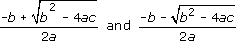for the solutions of the equation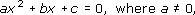we find that the solutions of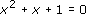are apparently given by the expressions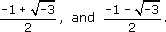These make no sense at all until we turn a blind eye to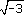and just manipulate it formally, as though we knew what we were doing, to give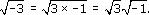We then say that if i2 = −1 then we might as well press on and replace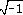by i and so the “solutions” of the equation are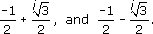We can check our formal manipulation of square root signs by substituting these “solutions” into the equation and see that they work. But before we can do that, we need to know how to “add” and “multiply” “numbers” of the form x + iy, where x and y are real. If we assume that they add and multiply according to the ordinary laws of algebra, in other words that addition and multiplication are associative and commutative and that multiplication is distributive over addition, then we have (a  + ib ) + ( c  +  id ) = ( a  + c)  + i( b + d ) and (a  + ib ) × (c  + id ) = ac  + i ( bc  + ad )  + i2bd.

If we replace i2 by −1, we obtain (a  + ib) × (c  + id) = ( acbd )  + i (bc  + ad ).

Now you can substitute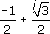into x2  + x  +  1 and see that our formal juggling with square root signs appears to be justified.

In the same way, we can factorise formally the expression x2 + bx + c into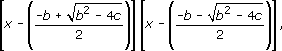and hence “solve” any quadratic equation by assuming also that the property αβ = 0 if and only if α = 0 or β = 0, which does apply to R, carries over to our new system. (Note the use of “or” in the “or both” sense.)

It may disconcert you to see us play around with a symbol that behaves as though it were the square root of −1: it should because, as yet, we have given no formal definition of this symbol, i. The problem that Pythagoras had in relating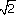to the set of rationals is presumably similar to that which we have in relating i to the set of reals. Just as we feel more comfortable when we are able to define irrational numbers in terms of rational numbers, so we must define numbers of the form a + ib in terms of the real numbers and justify our somewhat suspect manipulations. First we make the formal definition: then we deduce that our manipulations are in order.

It is easy to do this once you realise that a + ib is completely specified by the ordered pair (a, b) of real numbers (putting aside for the moment the meaning of the symbol i) and so the set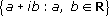can be put into one-one correspondence with R × R. To turn this correspondence into an isomorphism, we need to define suitable addition and multiplication operations on R × R. Keeping an eye on the operations we have already found useful, we make the following definition.

## Definition

The complex number system, denoted by C, is the set of all ordered pairs of real numbers (that is, R × R) with the operations of addition (denoted by +) and multiplication (denoted by ×) which satisfy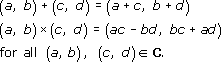It is straightforward but tedious to check that + and × defined as above are both commutative and associative and that × is distributive over +.

These definitions make perfect sense for any real numbers a, b, c and d and present no logical problems about square roots of −1 (no such square roots being mentioned in the definition). Thus we have turned round the earlier process and have defined a complex number as an ordered pair of real numbers which obeys suitable operations + and ×.

The notion of equality of complex numbers, comes from equality in R × R.

Two complex numbers, (a, b) and (c, d) are equal if and only if a = c and b = d.

Having made a proper definition of complex numbers, we now go back and restore our original notation, which, as we shall see, is far more convenient than the ordered pair notation. To do this, we note first of all that the subset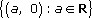of C is isomorphic to R, with the complex number (a, 0) corresponding to the real number a. (“A is isomorphic to B” means that there is an isomorphism from A onto B.) It is obviously more convenient to write a instead of (a, 0) and furthermore, because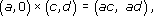we can write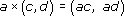or, more concisely,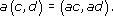Then any complex number, (a, b), can be written as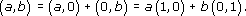We have already identified (1, 0) with the real number 1; all that remains is to replace (0, 1) by a symbol, i, of course. Thus the symbols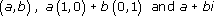represent the same complex number, and we write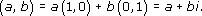## Definition

The symbol i represents (0, 1)C.

You may have noticed that we have just written a + bi instead of a + ib. It does not matter which you use.

As we remarked earlier, addition and multiplication of complex numbers (regarded as elements of R × R) are commutative and associative, and multiplication is distributive over addition. Numbers of the form a + ib can therefore be manipulated according to the ordinary laws of algebra to give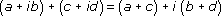and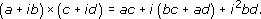We now have to interpret the symbol i2. It corresponds to (0, 1) × (0, 1) and direct application of the definition of multiplication shows that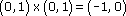which we are now abbreviating to −1. We are thus able to write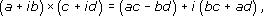which corresponds to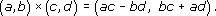Let us summarise these manoeuvres. Practical considerations suggest that a “number”, i, with the property i2 = −1 might be very useful. But symbols such asobviously present logical difficulties. Nevertheless, brushing aside these difficulties, we can predict how numbers a + bi would behave. We use this information to define a perfectly logical structure on R × R that is isomorphic to the system we are trying to construct. By taking this structure on R × R as a starting point we are able to identify i with the ordered pair (0, 1) and a + bi with the ordered pair (a, b). The notation a + bi is thus nothing more than an alternative form for (a, b): its advantage is that expressions like (a  + bi ) × ( c  + di) can be manipulated according to the ordinary laws of algebra with i2 replaced by −1 whenever it appears.

It is often necessary to refer to the first and second components of a complex number and so they are given names.

## Definition

The real number a is called the real part of a+ ib and the real number b is called the imaginary part of a + ib.

Notation

We shall write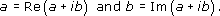Conventions

A complex number which has imaginary part zero is often called “real”, because even though it is not a real number it is, as we have seen, so closely identified with a real number that to insist on the distinction would be pedantic.

We shall find it useful to denote complex numbers by single letters. In particular, just as in real analysis x is often used to denote a variable real number, so, in complex analysis, z is a popular choice of symbol to denote a variable complex number. Furthermore, we shall nearly always implicitly assume that x and y are real and that x, y and z are related by the equations: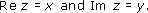In other words,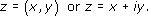It is interesting to notice that since two complex numbers are equal if and only if their real parts are equal and their imaginary parts are equal, a single equation connecting complex numbers leads to two equations connecting real numbers. Thus if w and z are complex numbers, we can deduce from the single equation

w = z

that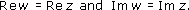This process is usually referred to as equating real and imaginary parts.

As you must have realised, despite its name, the imaginary part of a complex number is a perfectly ordinary real number. The name is an unfortunate one because it often leads to the mistaken impression that the imaginary part of a + ib is ib rather than b. The term “imaginary” arises from the original concern about complex numbers associated with the symbol. The way we have defined it, i is no more imaginary than the number.

Now that we all know what a complex number is, we need to agree on a list of properties of C that we can take as a starting point for complex analysis. We already know that C has some of the properties of R (such as associativity and commutativity of addition and of multiplication, and distributivity of multiplication over addition). In fact, all the familiar arithmetic (field) properties of R carry over to C as theorems, in the sense that they can be proved from the properties of R. (The concept of a field is discussed fully in M. Spivak, Calculus. A field is a set with two closed binary operations (+ and ×) for which properties (i)–(ix) are satisfied.) In the list that follows z,z1,z2,z3 are any elements of C: in other words the properties hold for all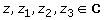.

Properties of C

• (i) Associative law for addition:

•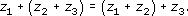• (ii) Existence of an additive identity (denoted by 0):

•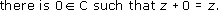• (iii) Existence of additive inverses:

•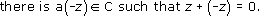• (iv) Commutative law for addition:

•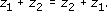• (v) Associative law for multiplication:

•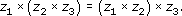• (vi) Existence of a multiplicative identity (denoted by 1):

•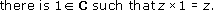• (vii) Existence of multiplicative inverses of non-zero numbers:

•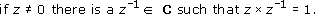• (viii) Commutative law for multiplication:

•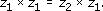• (ix) Distributive law:

•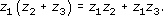(Note that in (ix) we have suppressed the multiplication symbol. This is common practice, as is the use of · for ×.)

We have already mentioned properties (i), (iv), (v), (viii) and (ix). Properties (ii), (iii), (vi) and (vii) are easily checked.

The additive identity, 0, is (0, 0) = 0 + 0i and the additive inverse of z = x + iy is −x + i(−y) which is written −xiy or −z. As with the real numbers, we define the difference of two complex numbers by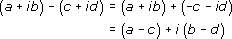or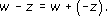The multiplicative identity, 1, is (1, 0) = 1 + 0i, and so if w = u + iv is the multiplicative inverse of z = x + iy then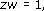that is,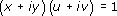and so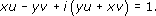Thus, equating real and imaginary parts, we have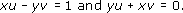giving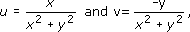since x and y cannot both be zero (because z ≠ 0). Thus the multiplicative inverse of x + iy is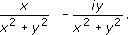All the other useful facts about C, such as the uniqueness of 0, wz = 0 if and only if w = 0 or z = 0, and so on, follow from the nine properties as for real numbers. The importance of this is that all the usual identities, such as the difference of two squares, the sum of a (finite) geometric series, the binomial theorem for a positive integer index go through as in real analysis. But now we come to the differences between real analysis and complex analysis. Most real analysis courses rely heavily on other properties of the real numbers – properties that tell us that the real numbers are ordered and enable us to define inequalities on the real numbers. (See M. Spivak, Calculus, pages 9 and 10.) This is a rather special use of the term “ordered”. We talk of a field being ordered if we can identify a subset of “positive elements’ such that one and only one of the following holds for each element, a, of the field: a is positive; −a is positive; a is zero (the trichotomy law) and, if a and b are positive then so are a + b and a × b. (The set of real numbers is an example of an ordered fieId.) In this sense of the word “ordered”, clearly there are problems with trying to turn C into an ordered field. For how could we define “positive” and “negative” subsets in C which have the properties we would expect?

The concept of an ordered field is an attempt to characterise the special ordering of the real numbers and it is fairly clear that the two-dimensional nature of the complex numbers is going to rule out the possibility of their being ordered in this sense. But suspicion is not enough; we should prove that C is not an ordered field.

It is easy enough to show that in any structure satisfying (i)–(ix) and in which we can talk of “positive” and “negative” elements, the square of any non-zero element is positive. This is precisely the property we set out not to have in constructing the complex numbers! (For, if a is positive then a × a = a2 must be positive, by the trichotomy law, and if a is negative then −a is positive and so (−a) × (−a) = a2 is positive, again by the trichotomy law.) If we wanted in some way to extend the concepts of positive and negative from R to C we would presumably require (1, 0) to be positive, in which case, by the trichotomy law, (−1, 0) would be negative. Then (0, 1) is non-zero, but (0, 1)2 = (−1, 0) is negative (and so we have the square of a non-zero element being negative). If we decided to get round this difficulty by calling (−1, 0) “positive”, it follows that −(−1, 0) = (1, 0) must be non-zero: also, then (1, 0)2 = (1, 0) is negative (and again we have the square of a non-zero element being negative). We have shown that it is not possible to find two classes which we could justifiably call “positive” and “negative”. It was precisely the existence of these two classes in R that enabled us to define inequalities in R.

We therefore come to the conclusion that the relation < does not carry over from the real numbers to the complex numbers.

It is precisely the relation < that enables us to talk of least upper bounds (see Spivak) – and so in moving from real analysis to complex analysis we have to throw overboard the least upper bound property that is so useful in real analysis.

Fortunately things are not as black as they might seem, because the proofs in which least upper bounds are used most frequently in real analysis can also be done by the use of the technique by repeated bisection. This relies on a theorem (proved from the least upper bound property) called the Nested Intervals Theorem (see Spivak). Happily, this theorem has an analogue in complex analysis called the Nested Rectangles Theorem. We shall prove this theorem in Section 4.

M332_1

### Take your learning further

Making the decision to study can be a big step, which is why you'll want a trusted University. The Open University has 50 years’ experience delivering flexible learning and 170,000 students are studying with us right now. Take a look at all Open University courses.

If you are new to university level study, find out more about the types of qualifications we offer, including our entry level Access courses and Certificates.

Not ready for University study then browse over 900 free courses on OpenLearn and sign up to our newsletter to hear about new free courses as they are released.

Every year, thousands of students decide to study with The Open University. With over 120 qualifications, we’ve got the right course for you.

Request an Open University prospectus